# 余弦退火调整学习率 CosineAnnealingLR

### CosineAnnealingLR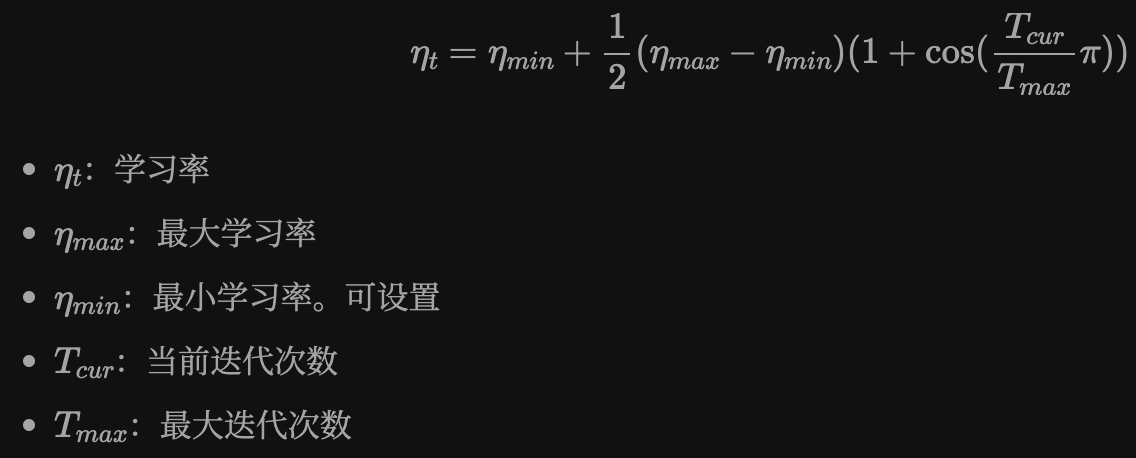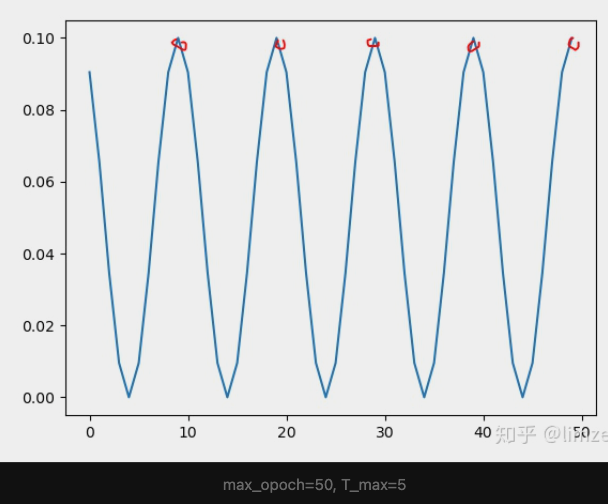### CosineAnnealingWarmRestarts

class torch.optim.lr_scheduler.CosineAnnealingWarmRestarts(optimizer, T_0, T_mult=1, eta_min=0, last_epoch=-1)

• optimizer：优化器
• T_0：重启后的迭代次数
• T_mult：每次重启后增加迭代次数的乘法因子。默认为 1
• eta_min：最小学习率。默认为 0
• last_epoch：最新一轮。默认为 -1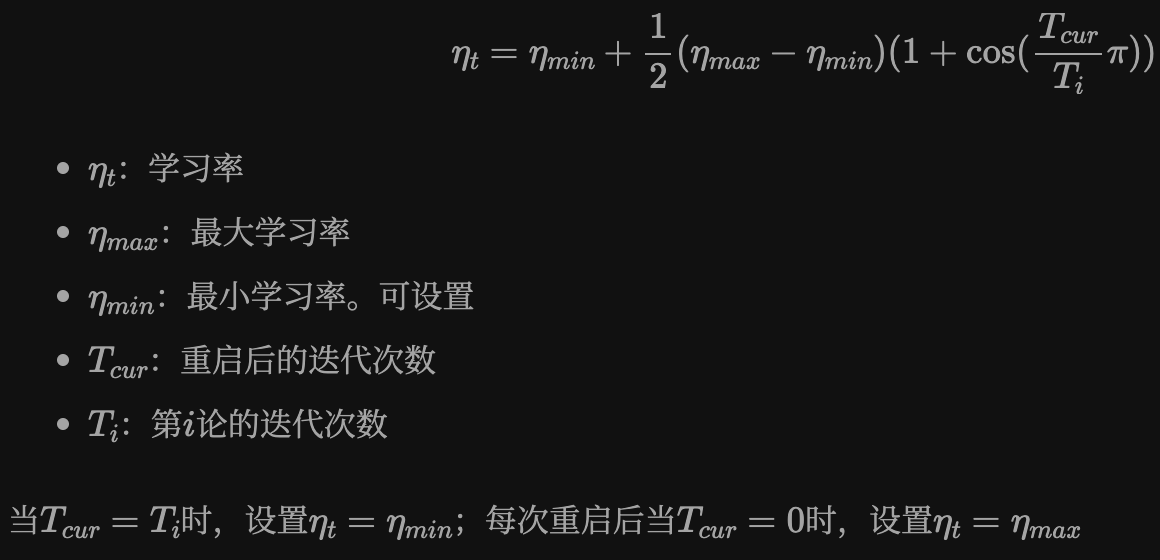• T_0: 学习率第一次回到初始值的 epoch 位置
• T_mult: 这个控制了学习率变化的速度, 重启之后因子，默认是 1
• 每次 restart 后，T_0 = T_0 * T_mult
• 如果 T_mult=1, 则学习率在 T_0, 2T_0, 3T_0, ...., i*T_0, .... 处回到最大值(初始学习率)
• 5, 10, 15, 20, 25, ....... 处回到最大值
• 如果 T_mult>1, 则学习率在 T_0, (1+T_mult)T_0,(1+T_mult+T_mult**2)T_0, ....., (1+T_mult+T_mult2+...+T_0i)*T0, 处回到最大值
• 5, 15, 35, 75, 155, ....... 处回到最大值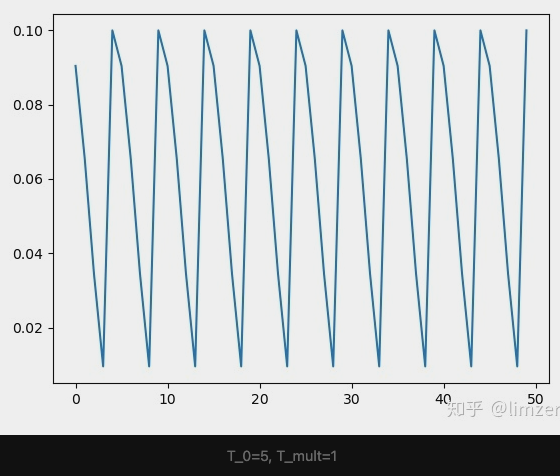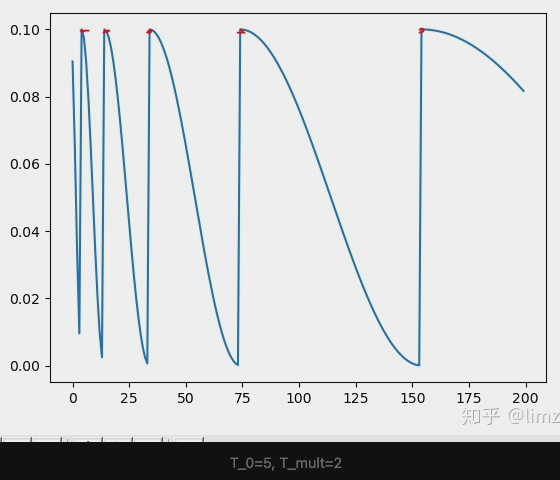### 使用示例

```import torch
from torch.optim.lr_scheduler import CosineAnnealingLR, CosineAnnealingWarmRestarts, StepLR
import torch.nn as nn
from torchvision.models import resnet18
import matplotlib.pyplot as plt

model = resnet18(pretrained=False)
optimizer = torch.optim.SGD(model.parameters(), lr=0.1)
mode = 'cosineAnnWarm'
if mode == 'cosineAnn':
scheduler = CosineAnnealingLR(optimizer, T_max=5, eta_min=0)
elif mode == 'cosineAnnWarm':
scheduler = CosineAnnealingWarmRestarts(optimizer, T_0=5, T_mult=1)
'''
以 T_0=5, T_mult=1 为例:
T_0:学习率第一次回到初始值的 epoch 位置.
T_mult: 这个控制了学习率回升的速度
- 如果 T_mult=1, 则学习率在 T_0, 2*T_0, 3*T_0, ...., i*T_0, .... 处回到最大值(初始学习率)
- 5,10,15,20,25,.......处回到最大值
- 如果 T_mult>1, 则学习率在 T_0, (1+T_mult)*T_0, (1+T_mult+T_mult**2)*T_0, ....., (1+T_mult+T_mult**2+...+T_0**i)*T0, 处回到最大值
- 5,15,35,75,155,.......处回到最大值
example:
T_0=5, T_mult=1
'''
plt.figure()
max_epoch = 50
iters = 200
cur_lr_list = []
for epoch in range(max_epoch):
for batch in range(iters):
'''
这里 scheduler.step(epoch + batch / iters) 的理解如下, 如果是一个 epoch 结束后再 .step
那么一个 epoch 内所有 batch 使用的都是同一个学习率, 为了使得不同 batch 也使用不同的学习率
则可以在这里进行 .step
'''
#scheduler.step(epoch + batch / iters)
optimizer.step()
scheduler.step()
cur_lr = optimizer.param_groups[-1]['lr']
cur_lr_list.append(cur_lr)
print('cur_lr:',cur_lr)
x_list = list(range(len(cur_lr_list)))
plt.plot(x_list, cur_lr_list)
plt.show()```
```import torch
from torch.optim.lr_scheduler import CosineAnnealingLR, CosineAnnealingWarmRestarts, StepLR
import torch.nn as nn
from torchvision.models import resnet18
import matplotlib.pyplot as plt

model = resnet18(pretrained=False)
optimizer = torch.optim.SGD(model.parameters(), lr=0.1)
mode = 'cosineAnnWarm'
if mode == 'cosineAnn':
scheduler = CosineAnnealingLR(optimizer, T_max=5, eta_min=0)
elif mode=='cosineAnnWarm':
scheduler = CosineAnnealingWarmRestarts(optimizer, T_0=2, T_mult=2)
plt.figure()
max_epoch = 20
iters = 5
cur_lr_list = []
for epoch in range(max_epoch):
print('epoch_{}'.format(epoch))
for batch in range(iters):
scheduler.step(epoch + batch / iters)
optimizer.step()
# scheduler.step()
cur_lr = optimizer.param_groups[-1]['lr']
cur_lr_list.append(cur_lr)
print('cur_lr:', cur_lr)
print('epoch_{}_end'.format(epoch))
x_list = list(range(len(cur_lr_list)))
plt.plot(x_list, cur_lr_list)
plt.show()```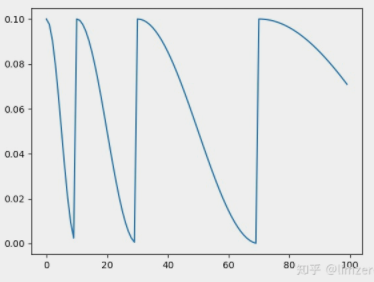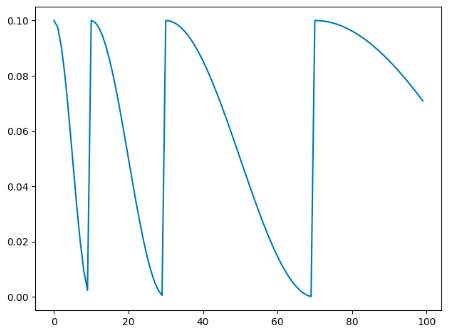### reference

https://zhuanlan.zhihu.com/p/261134624